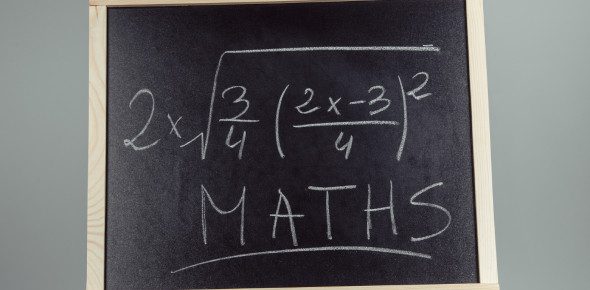# Ultimate Quiz On Factoring Polynomial

10 Questions | Attempts: 666
ShareSettingsHow good are you at factorizing polynomials? Let's test it with the ultimate quiz on factoring polynomials. In mathematics and computer algebra, polynomial factorization expresses in the integers as the product of irreducible factors with coefficients in the same domain. If you wish to practice factorization of polynomials, it is a perfect quiz for you. Go for it, and check out how much you score. After that, you can share the quiz results with others and challenge them on factorization.

• 1.
What is the greatest common factor of the polynomial 8x+14x–32?
• A.

2x

• B.

2

• C.

4

• D.

8

• 2.
Name the greatest common factor of the terms of the binomial –24x2–18x
• A.

–6x

• B.

–8x

• C.

2x

• D.

6

• 3.
Which shows the polynomial fully factored? 35x2 – 14x
• A.

–7x(5x + 2)

• B.

7(5x^2–2x)

• C.

7(5x–2x)

• D.

7x(5x–2)

• 4.
Which shows the polynomial fully factored? 2x(4x–3) – 5(4x – 3)
• A.

(4x – 3)(2x+5)

• B.

(8x^2–6x)–(20x–15)

• C.

(4x–3)(2x–5)

• D.

–10x(4x–3)

• 5.
Which shows the missing factor? 12b2 – 72b = 12b(?)
• A.

B^2 – 6b

• B.

–b + 6

• C.

6b

• D.

B – 6

• 6.
A parallelogram has a height of x+3 and an area of 25x2+75x.  What is the measure of the base?
• A.

5x^2

• B.

25x

• C.

X+25

• D.

25

• 7.
A square has an area of 25x2 +30x+ 9.  What is the measure of one side of the square?
• A.

X+3

• B.

5x+1

• C.

5x+3

• D.

5x+9

• 8.
Kevin is designing a label for a can.  The height of the label is 5cm.  If the area of the label is 10n2+20n–5, how long is the label?
• A.

2n^2+4n–1

• B.

N^2+2n–1

• C.

2n^2–4n+1

• D.

2n^2+5n–1

• 9.
The formula for the surface area of a sphere is SA = 4πr2.  If the surface area of the sphere is 4πx^2–8πx+4π, what is the measure of the radius?
• A.

X+1

• B.

2x–1

• C.

X–2

• D.

X–1

• 10.
Classify the following equation: 8x2–4x+9
• A.

Trinomial

• B.

Monomial

• C.

Binomial

• D.Back to top